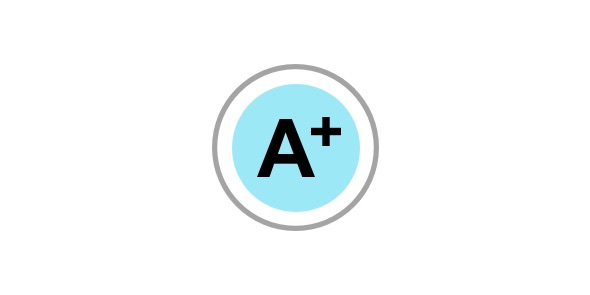G. Science (Grade 5 - 8)

26 Questions | Total Attempts: 515Settings• 1.
What do you know about hereditary diseases? Comment how they are transferred from parents to offsprings?
• 2.
Write briefly about the following: Plaster of Paris: Reflex Action:
• 3.
Which techniques you will use to motivate the students?
• 4.
What would you do if the whole class is not getting the concept?
• 5.
How can you make learning fun for the students?
• 6.
Why should we consider you for the teaching job in our school?
• 7.
Water is heated in a kettle. The inside water is heated by convection. A person sitting near the fire receives heat by.
• A.

Conduction

• B.

Convection

• C.

• D.

Reflection

• 8.
Specific gravity of milk is measured by an instrument known as:
• A.

Lactometer

• B.

Barometer

• C.

Hydrometer

• D.

Pedometer

• 9.
Light year is a unit of
• A.

Distance

• B.

Time period

• C.

Light intensity

• D.

Time

• 10.
Three elements needed for the healthy growth of plants are
• A.

N, P, K

• B.

N, C, P

• C.

N, K, C

• D.

N, S, P

• 11.
Copper can be converted into gold by:
• A.

Heating

• B.

• C.

Electroplating

• D.

Chemical Reaction

• 12.
The echo (reflected sound) will be distinctly heard only at ordinary temperatures if the distance of the reflecting surfaces from the source of sound is at least:
• A.

13 m

• B.

17 m

• C.

19 m

• D.

23 m

• 13.
When a ray of sunlight enters a dark room, its straight path becomes visible because of dustparticles hanging in the air. It is because light is:
• A.

Visible

• B.

Transparent

• C.

Invisible

• D.

Opaque

• 14.
The temperature of the dead body is:
• A.

Temperature of the place where it is kept

• B.

37

• C.

Room temperature

• D.

0

• 15.
Cusec is a unit of:
• A.

Rate of flow

• B.

Time

• C.

Distance

• D.

Mass

• 16.
The deepest place on earth is:
• A.

Trench

• B.

Mariana Trench

• C.

Mangrove

• D.

Groove

• 17.
There are __________ parts of small intestine
• A.

2

• B.

3

• C.

4

• D.

5

• 18.
Bronze medal is made up of metals:
• A.

Copper, Nickel

• B.

Copper, Tin

• C.

Copper, Silver

• D.

Copper, Zinc

• 19.
Which of the following, lists the four stages of food processing in order?
• A.

Ingestion, digestion, absorption, elimination

• B.

Digestion, ingestion, absorption, elimination

• C.

Ingestion, absorption, elimination, digestion

• D.

Absorption, digestion, ingestion, elimination

• 20.
Cobalt is a material which is
• A.

Strongly attracted by a magnet

• B.

Not attracted by a magnet

• C.

Not a magnet

• D.

Weakly attracted by a magnet

• 21.
Hepatitis A is transmitted to different individuals by:
• A.

Water

• B.

Sneezing

• C.

Spit

• D.

Faeces

• 22.
The most abundant element present in the human body is:
• A.

Nitrogen

• B.

Carbon

• C.

Hydrogen

• D.

Oxygen

• 23.
Cancer can be treated by:
• A.

Antibiotics and vaccines

• B.

• C.

• D.

Antibodies and chemotherapy

• 24.
There are ___________________ planets in our solar system.
• A.

Six

• B.

Seven

• C.

Eight

• D.

Nine

• 25.
A time can come when we will be able to design a machine which can go on working for ever without the expenditure of energy. Is it possible?
• A.

No

• B.

Yes

• C.

In due course of time

• D.

None of the above

Related TopicsBack to top